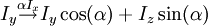# Drawing mathematical equations

(Difference between revisions)
 Revision as of 20:55, 2 September 2008 (edit)← Previous diff Current revision (23:29, 26 January 2009) (edit) (undo) Line 8: Line 8: '''Many more examples''' are available [http://meta.wikimedia.org/wiki/Help:Formula#Larger_Expressions here] '''Many more examples''' are available [http://meta.wikimedia.org/wiki/Help:Formula#Larger_Expressions here] + + [[Category:NMRWiki Help]]

## Current revision

Place latex code between  tags

Here is an example

$I_y \stackrel{\alpha I_x}{\rightarrow} I_y \cos(\alpha) + I_z\sin(\alpha)$


which gives$I_y \stackrel{\alpha I_x}{\rightarrow} I_y \cos(\alpha) + I_z\sin(\alpha)$

Many more examples are available here